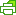# NUMERICAL TECHNIQUE FOR SOLVING TRUSS AND PLANE PROBLEMS FORA NEW CLASS OF ELASTIC BODIES

Author: S Rajthilak, L S Shankar, U Saravanan

Organization: Indian Institute of Technology Madras

Mail Adress: [email protected]

Abstract: It is customary to use displacement based formulation to seek solution to boundary value problems for its computational efficacy. In displacement based formulations, it is convenient to prescribe the constitutive relation for stress as an explicit function of displacement gradient. However, from a general theoretical point of view, the stress and displacement gradient could be related through an implicit function. In this view point, a special case is one where the linearized strain is related to the stress explicitly. This study develops techniques to solve boundary value problems when linearized strain is written as an explicit function of the stress. Here both the stresses and the displacement are taken as unknowns. Stress field is constructed such that it satisfies the equilibrium equations identically within the element and the traction continuity requirements between the elements. Continuously differentiable displacement field is constructed and the linearized strain computed from this displacement field. Then, the unknown parameters in the stress and displacement field are estimated such that the difference between the strain computed from the constitutive relation for the assumed stress field and the strain estimated from the assumed displacement field are close in some sense. Though in this procedure the number of unknowns has increased in comparison to the displacement formulation, both the strength and serviceability condition can be checked directly without any post processing. Also, in this procedure, both the equilibrium equations and continuity of displacement are met exactly. The equation that is not satisfied exactly is the constitutive relation, which is an approximation anyway. The efficacy and accuracy of this method is bench marked by studying some standard problems. Planar and three dimensional truss elements to analyze trusses has been developed and bench marked. Then, two dimensional plane strain and stress element is implemented and its performance recorded.PrintPDF